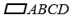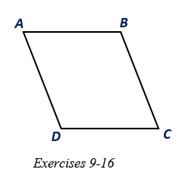Chapter 4.1, Problem 11EElementary Geometry For College St...

7th Edition
Alexander + 2 others
ISBN: 9781337614085

Solutions

Chapter
SectionElementary Geometry For College St...

7th Edition
Alexander + 2 others
ISBN: 9781337614085
Textbook Problem

Given that m ∠ A = 2 x + 3 , and m ∠ B = 3 x - 23 , find the measure of each angle of.To determine

To find:

The measure of each angle of ABCD.

Explanation

Given:

Given that mA=2x+3, and mB=3x-23, and the ABCD as shown below.

Corollary:

1. The opposite angles of a parallelogram are congruent.

2. Two consecutive angles of a parallelogram are supplementary.

Calculation:

In the given parallelogram ABCD, given that mA=2x+3, and mB=3x-23.

As per the corollary that the two consecutive angles of a parallelogram are supplementary, the sum of the angles mA and mB is 180°.

To find the value of x, substitute mA=2x+3, and mB=3x-23 in,

mA+mB=180°

We have,

2x+3+3x-23=180°

5x-20=180°

5x=180°+20°

5x=200°

x=20005

x=40°

To find mA, substitute x =40 in mA=2x<

Still sussing out bartleby?

Check out a sample textbook solution.

See a sample solution

The Solution to Your Study Problems

Bartleby provides explanations to thousands of textbook problems written by our experts, many with advanced degrees!

Get Started

In Exercises 63-68, use the graph of the function f to determine limxf(x) and limxf(x) 65.

Applied Calculus for the Managerial, Life, and Social Sciences: A Brief Approach

What is the value of (X + 1) for the following scores: 0, 1, 4, 2? a. 8 b. 9 c. 11 d. 16

Essentials of Statistics for The Behavioral Sciences (MindTap Course List)

Use two disjoint sets to show that 0+2=0.

Mathematical Excursions (MindTap Course List)

True or False: The x-intercepts are the values of x for which f(x) = 0.

Study Guide for Stewart's Single Variable Calculus: Early Transcendentals, 8th